### Home > MC1 > Chapter 4 > Lesson 4.3.5 > Problem4-130

4-130.
1. . In this lesson you looked for ways to convert between equivalent forms of fractions, decimals, and percents. For each of the given portions, write the other forms of the number. Show your work so that a team member could understand your process. Homework Help ✎

1. Write

2.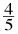as a decimal and as a percent.

3. Write 0.30 as a fraction and as a percent.

4. Write 85% as a fraction and as a decimal.

5. Write 1.23 as a percent and as a fraction.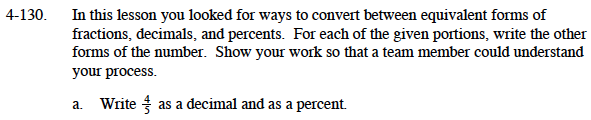If you have trouble with percents, refer to the ''Useful Percents to Remember''
section of the Math Notes box from Lesson 4.3.5 below.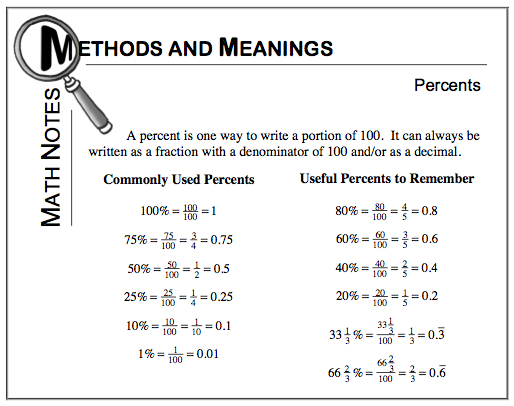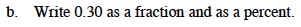This can be said as 3-tenths and 0-hundredths or 30 hundredths. How can this help you rewrite it?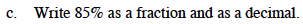How is this problem similar to the commonly used percent shown below?

$75\%\;=\;\frac{75}{100}\;=\;\frac{3}{4}\;=\;0.75$

$85\%\text{ is equal to }\frac{85}{100}\text{ and }0.85.$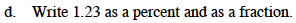$\text{We know that 1 is equal to: }100\%\text{ and }\frac{100}{100}.$

Can you find what percent and fraction are equal to 0.23? Then, you will need to add the two values.

1.23 is equal to %123